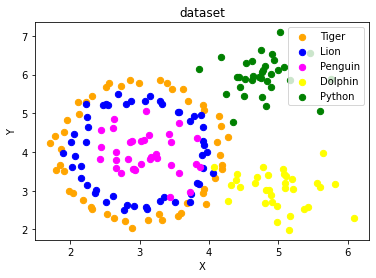## Data Generation

### Generate Synthetical Data with Python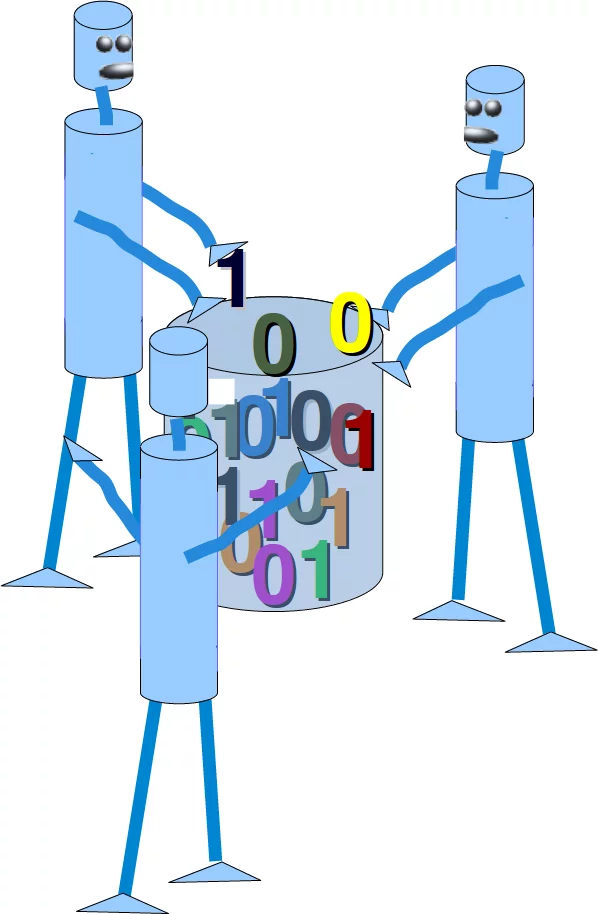A problem with machine learning, especially when you are starting out and want to learn about the algorithms, is that it is often difficult to get suitable test data. Some cost a lot of money, others are not freely available because they are protected by copyright. Therefore, artificially generated test data can be a solution in some cases.

For this reason, this chapter of our tutorial deals with the artificial generation of data. This chapter is about creating artificial data. In the previous chapters of our tutorial we learned that Scikit-Learn (sklearn) contains different data sets. On the one hand, there are small toy data sets, but it also offers larger data sets that are often used in the machine learning community to test algorithms or also serve as a benchmark. It provides us with data coming from the 'real world'.

All this is great, but in many cases this is still not sufficient. Maybe you find the right kind of data, but you need more data of this kind or the data is not completely the kind of data you were looking for, e.g. maybe you need more complex or less complex data. This is the point where you should consider to create the data yourself. Here, sklearn offers help. It includes various random sample generators that can be used to create custom-made artificial datasets. Datasets that meet your ideas of size and complexity.

The following Python code is a simple example in which we create artificial weather data for some German cities. We use Pandas and Numpy to create the data:

import numpy as np
import pandas as pd

cities = ['Berlin', 'Frankfurt', 'Hamburg',
'Nuremberg', 'Munich', 'Stuttgart',
'Hanover', 'Saarbruecken', 'Cologne',
'Constance', 'Freiburg', 'Karlsruhe'
]

n= len(cities)
data = {'Temperature': np.random.normal(24, 3, n),
'Humidity': np.random.normal(78, 2.5, n),
'Wind': np.random.normal(15, 4, n)
}
df = pd.DataFrame(data=data, index=cities)
df

Output:
Temperature Humidity Wind
Berlin 26.133572 80.328353 5.015542
Frankfurt 23.161901 76.886831 14.021860
Hamburg 24.145767 79.678764 10.897413
Nuremberg 27.114319 77.825100 21.273423
Munich 24.573419 74.416920 21.734649
Stuttgart 20.615747 81.827868 6.316270
Hanover 26.513550 80.462603 9.568481
Saarbruecken 23.602173 83.181582 11.382041
Cologne 27.769321 79.759665 11.433353
Constance 29.343985 78.814028 17.818053
Freiburg 25.554123 77.339895 7.965502
Karlsruhe 19.780618 76.517790 6.304491

### Another Example

We will create artificial data for four nonexistent types of flowers. If the names remind you of programming languages and pizza, it will be no coincidence:

• Flos Pythonem
• Flos Java
• Flos Margarita
• Flos artificialis

The RGB avarage colors values are correspondingly:

• (255, 0, 0)
• (245, 107, 0)
• (206, 99, 1)
• (255, 254, 101)

The average diameter of the calyx is:

• 3.8
• 3.3
• 4.1
• 2.9
Flos pythonem
(254, 0, 0)
Flos Java
(245, 107, 0)
Flos margarita
(206, 99, 1)
Flos artificialis
(255, 254, 101)
import matplotlib.pyplot as plt
import numpy as np
import pandas as pd

from scipy.stats import truncnorm

def truncated_normal(mean=0, sd=1, low=0, upp=10, type=int):
return truncnorm(
(low - mean) / sd, (upp - mean) / sd, loc=mean, scale=sd)

def truncated_normal_floats(mean=0, sd=1, low=0, upp=10, num=100):
res = truncated_normal(mean=mean, sd=sd, low=low, upp=upp)
return res.rvs(num)

def truncated_normal_ints(mean=0, sd=1, low=0, upp=10, num=100):
res = truncated_normal(mean=mean, sd=sd, low=low, upp=upp)
return res.rvs(num).astype(np.uint8)

# number of items for each flower class:
number_of_items_per_class = [190, 205, 230, 170]
flowers = {}
# flos Pythonem:
number_of_items = number_of_items_per_class
reds = truncated_normal_ints(mean=254, sd=18, low=235, upp=256,
num=number_of_items)
greens = truncated_normal_ints(mean=107, sd=11, low=88, upp=127,
num=number_of_items)
blues = truncated_normal_ints(mean=0, sd=15, low=0, upp=20,
num=number_of_items)
calyx_dia = truncated_normal_floats(3.8, 0.3, 3.4, 4.2,
num=number_of_items)
data = np.column_stack((reds, greens, blues, calyx_dia))
flowers["flos_pythonem"] = data

# flos Java:
number_of_items = number_of_items_per_class
reds = truncated_normal_ints(mean=245, sd=17, low=226, upp=256,
num=number_of_items)
greens = truncated_normal_ints(mean=107, sd=11, low=88, upp=127,
num=number_of_items)
blues = truncated_normal_ints(mean=0, sd=10, low=0, upp=20,
num=number_of_items)
calyx_dia = truncated_normal_floats(3.3, 0.3, 3.0, 3.5,
num=number_of_items)
data = np.column_stack((reds, greens, blues, calyx_dia))
flowers["flos_java"] = data

# flos Java:
number_of_items = number_of_items_per_class
reds = truncated_normal_ints(mean=206, sd=17, low=175, upp=238,
num=number_of_items)
greens = truncated_normal_ints(mean=99, sd=14, low=80, upp=120,
num=number_of_items)
blues = truncated_normal_ints(mean=1, sd=5, low=0, upp=12,
num=number_of_items)
calyx_dia = truncated_normal_floats(4.1, 0.3, 3.8, 4.4,
num=number_of_items)
data = np.column_stack((reds, greens, blues, calyx_dia))
flowers["flos_margarita"] = data

# flos artificialis:
number_of_items = number_of_items_per_class
reds = truncated_normal_ints(mean=255, sd=8, low=2245, upp=2255,
num=number_of_items)
greens = truncated_normal_ints(mean=254, sd=10, low=240, upp=255,
num=number_of_items)
blues = truncated_normal_ints(mean=101, sd=5, low=90, upp=112,
num=number_of_items)
calyx_dia = truncated_normal_floats(2.9, 0.4, 2.4, 3.5,
num=number_of_items)
data = np.column_stack((reds, greens, blues, calyx_dia))
flowers["flos_artificialis"] = data

data = np.concatenate((flowers["flos_pythonem"],
flowers["flos_java"],
flowers["flos_margarita"],
flowers["flos_artificialis"]
), axis=0)

# assigning the labels
target = np.zeros(sum(number_of_items_per_class)) # 4 flowers
previous_end = 0
for i in range(1, 5):
num = number_of_items_per_class[i-1]
beg = previous_end
target[beg: beg + num] += i
previous_end = beg + num

conc_data = np.concatenate((data, target.reshape(target.shape, 1)),
axis=1)

np.savetxt("data/strange_flowers.txt", conc_data, fmt="%2.2f",)

import matplotlib.pyplot as plt

target_names = list(flowers.keys())
feature_names = ['red', 'green', 'blue', 'calyx']
n = 4
fig, ax = plt.subplots(n, n, figsize=(16, 16))

colors = ['blue', 'red', 'green', 'yellow']

for x in range(n):
for y in range(n):
xname = feature_names[x]
yname = feature_names[y]
for color_ind in range(len(target_names)):
ax[x, y].scatter(data[target==color_ind, x],
data[target==color_ind, y],
label=target_names[color_ind],
c=colors[color_ind])

ax[x, y].set_xlabel(xname)
ax[x, y].set_ylabel(yname)
ax[x, y].legend(loc='upper left')

plt.show()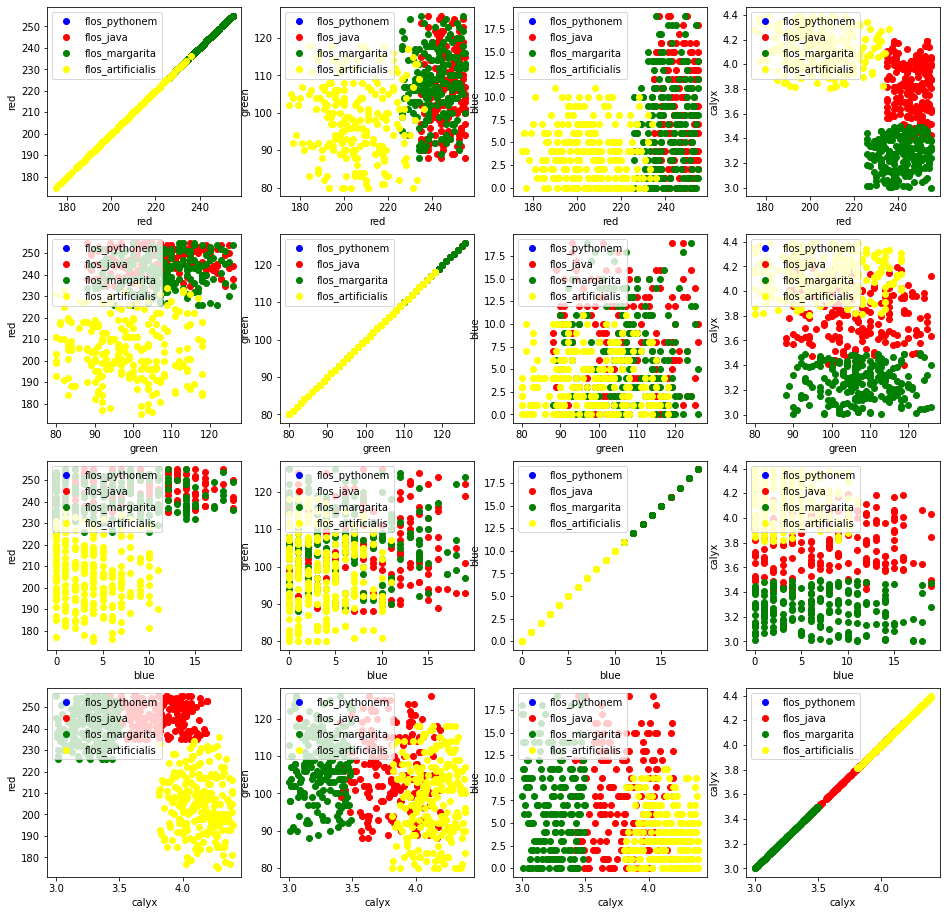### Generate Synthetic Data with Scikit-Learn

It is a lot easier to use the possibilities of Scikit-Learn to create synthetic data.

The functionalities available in sklearn can be grouped into

1. Generators for classifictation and clustering
2. Generators for creating data for regression
3. Generators for manifold learning
4. Generators for decomposition

#### Generators for Classification and Clustering

We start with the the function make_blobs of sklearn.datasets to create 'blob' like data distributions. By setting the value of centers to n_classes, we determine the number of blobs, i.e. the clusters. n_samples corresponds to the total number of points equally divided among clusters. If random_state is not set, we will have random results every time we call the function. We pass an int to this parameter for reproducible output across multiple function calls.

import numpy as np
import matplotlib.pyplot as plt
from sklearn.datasets import make_blobs

n_classes = 4
data, labels = make_blobs(n_samples=1000,
centers=n_classes,
random_state=100)

labels[:7]

Output:
array([1, 3, 1, 3, 1, 3, 2])

We will visualize the previously created blob custers with matplotlib:

fig, ax = plt.subplots()

colours = ('green', 'orange', 'blue', "pink")
for label in range(n_classes):
ax.scatter(x=data[labels==label, 0],
y=data[labels==label, 1],
c=colours[label],
s=40,
label=label)

ax.set(xlabel='X',
ylabel='Y',
title='Blobs Examples')

ax.legend(loc='upper right')

Output:
<matplotlib.legend.Legend at 0x7f1c3f9d0e80>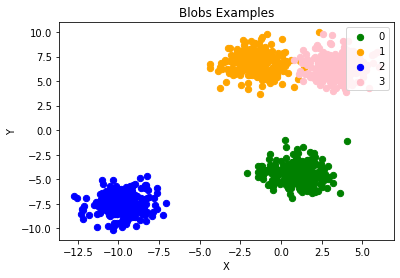The centers of the blobs were randomly chosen in the previous example. In the following example we set the centers of the blobs explicitly. We create a list with the center points and pass it to the parameter centers:

import numpy as np
import matplotlib.pyplot as plt
from sklearn.datasets import make_blobs

centers = [[2, 3], [4, 5], [7, 9]]
data, labels = make_blobs(n_samples=1000,
centers=np.array(centers),
random_state=1)

labels[:7]

Output:
array([0, 1, 1, 0, 2, 2, 2])

Let us have a look at the previously created blob clusters:

fig, ax = plt.subplots()

colours = ('green', 'orange', 'blue')
for label in range(len(centers)):
ax.scatter(x=data[labels==label, 0],
y=data[labels==label, 1],
c=colours[label],
s=40,
label=label)

ax.set(xlabel='X',
ylabel='Y',
title='Blobs Examples')

ax.legend(loc='upper right')

Output:
<matplotlib.legend.Legend at 0x7f1c3f363370>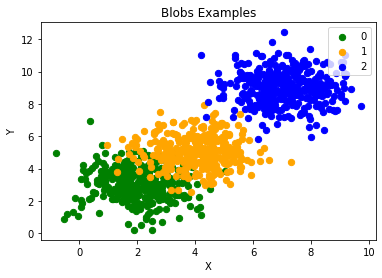Usually, you want to save your artificially created datasets in a file. For this purpose, we can use the function savetxt from numpy. Before we can do this we have to reaarange our data. Each row should contain both the data and the label:

import numpy as np

labels = labels.reshape((labels.shape,1))
all_data = np.concatenate((data, labels), axis=1)
all_data[:7]

Output:
array([[ 1.72415394,  4.22895559,  0.        ],
[ 4.16466507,  5.77817418,  1.        ],
[ 4.51441156,  4.98274913,  1.        ],
[ 1.49102772,  2.83351405,  0.        ],
[ 6.0386362 ,  7.57298437,  2.        ],
[ 5.61044976,  9.83428321,  2.        ],
[ 5.69202866, 10.47239631,  2.        ]])

For some people it might be complicated to understand the combination of reshape and concatenate. Therefore, you can see an extremely simple example in the following code:

import numpy as np

a = np.array( [[1, 2], [3, 4]])
b = np.array( [5, 6])
b = b.reshape((b.shape, 1))
print(b)

x = np.concatenate( (a, b), axis=1)
x

[
]

Output:
array([[1, 2, 5],
[3, 4, 6]])

We use the numpy function savetxt to save the data. Don't worry about the strange name, it is just for fun and for reasons which will be clear soon:

np.savetxt("squirrels.txt",
all_data,
fmt=['%.3f', '%.3f', '%1d'])
all_data[:10]

Output:
array([[ 1.72415394,  4.22895559,  0.        ],
[ 4.16466507,  5.77817418,  1.        ],
[ 4.51441156,  4.98274913,  1.        ],
[ 1.49102772,  2.83351405,  0.        ],
[ 6.0386362 ,  7.57298437,  2.        ],
[ 5.61044976,  9.83428321,  2.        ],
[ 5.69202866, 10.47239631,  2.        ],
[ 6.14017298,  8.56209179,  2.        ],
[ 2.97620068,  5.56776474,  1.        ],
[ 8.27980017,  8.54824406,  2.        ]])

## Reading the data and conversion back into 'data' and 'labels'

We will demonstrate now, how to read in the data again and how to split it into data and labels again:

file_data = np.loadtxt("squirrels.txt")

data = file_data[:,:-1]
labels = file_data[:,2:]

labels = labels.reshape((labels.shape))


We had called the data file squirrels.txt, because we imagined a strange kind of animal living in the Sahara desert. The x-values stand for the night vision capabilities of the animals and the y-values correspond to the colour of the fur, going from sandish to black. We have three kinds of squirrels, 0, 1, and 2. (Be aware that our squirrals are imaginary squirrels and have nothing to do with the real squirrels of the Sahara!)

import matplotlib.pyplot as plt

colours = ('green', 'red', 'blue', 'magenta', 'yellow', 'cyan')
n_classes = 3

fig, ax = plt.subplots()
for n_class in range(0, n_classes):
ax.scatter(data[labels==n_class, 0], data[labels==n_class, 1],
c=colours[n_class], s=10, label=str(n_class))

ax.set(xlabel='Night Vision',
ylabel='Fur color from sandish to black, 0 to 10 ',
title='Sahara Virtual Squirrel')

ax.legend(loc='upper right')

Output:
<matplotlib.legend.Legend at 0x7f1c3f2dfcd0>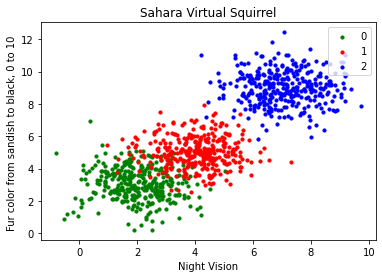We will train our articifical data in the following code:

from sklearn.model_selection import train_test_split

data_sets = train_test_split(data,
labels,
train_size=0.8,
test_size=0.2,
random_state=42 # garantees same output for every run
)

train_data, test_data, train_labels, test_labels = data_sets

# import model
from sklearn.neighbors import KNeighborsClassifier

# create classifier
knn = KNeighborsClassifier(n_neighbors=8)

# train
knn.fit(train_data, train_labels)

# test on test data:
calculated_labels = knn.predict(test_data)
calculated_labels

Output:
array([2., 0., 1., 1., 0., 1., 2., 2., 2., 2., 0., 1., 0., 0., 1., 0., 1.,
2., 0., 0., 1., 2., 1., 2., 2., 1., 2., 0., 0., 2., 0., 2., 2., 0.,
0., 2., 0., 0., 0., 1., 0., 1., 1., 2., 0., 2., 1., 2., 1., 0., 2.,
1., 1., 0., 1., 2., 1., 0., 0., 2., 1., 0., 1., 1., 0., 0., 0., 0.,
0., 0., 0., 1., 1., 0., 1., 1., 1., 0., 1., 2., 1., 2., 0., 2., 1.,
1., 0., 2., 2., 2., 0., 1., 1., 1., 2., 2., 0., 2., 2., 2., 2., 0.,
0., 1., 1., 1., 2., 1., 1., 1., 0., 2., 1., 2., 0., 0., 1., 0., 1.,
0., 2., 2., 2., 1., 1., 1., 0., 2., 1., 2., 2., 1., 2., 0., 2., 0.,
0., 1., 0., 2., 2., 0., 0., 1., 2., 1., 2., 0., 0., 2., 2., 0., 0.,
1., 2., 1., 2., 0., 0., 1., 2., 1., 0., 2., 2., 0., 2., 0., 0., 2.,
1., 0., 0., 0., 0., 2., 2., 1., 0., 2., 2., 1., 2., 0., 1., 1., 1.,
0., 1., 0., 1., 1., 2., 0., 2., 2., 1., 1., 1., 2.])
from sklearn import metrics

print("Accuracy:", metrics.accuracy_score(test_labels, calculated_labels))

Accuracy: 0.97


## Other Interesting Distributions

import numpy as np

import sklearn.datasets as ds
data, labels = ds.make_moons(n_samples=150,
shuffle=True,
noise=0.19,
random_state=None)

data += np.array(-np.ndarray.min(data[:,0]),
-np.ndarray.min(data[:,1]))

np.ndarray.min(data[:,0]), np.ndarray.min(data[:,1])

Output:
(0.0, 0.43385925898113253)
import matplotlib.pyplot as plt
fig, ax = plt.subplots()

ax.scatter(data[labels==0, 0], data[labels==0, 1],
c='orange', s=40, label='oranges')
ax.scatter(data[labels==1, 0], data[labels==1, 1],
c='blue', s=40, label='blues')

ax.set(xlabel='X',
ylabel='Y',
title='Moons')

#ax.legend(loc='upper right');

Output:
[Text(0.5, 0, 'X'), Text(0, 0.5, 'Y'), Text(0.5, 1.0, 'Moons')]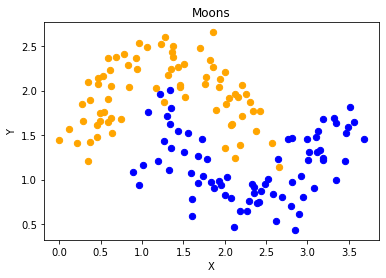We want to scale values that are in a range [min, max] in a range [a, b].

$$f(x) = \frac{(b-a)\cdot(x - min)}{max - min} + a$$

We now use this formula to transform both the X and Y coordinates of data into other ranges:

min_x_new, max_x_new = 33, 88
min_y_new, max_y_new = 12, 20

data, labels = ds.make_moons(n_samples=100,
shuffle=True,
noise=0.05,
random_state=None)

min_x, min_y = np.ndarray.min(data[:,0]), np.ndarray.min(data[:,1])
max_x, max_y = np.ndarray.max(data[:,0]), np.ndarray.max(data[:,1])

#data -= np.array([min_x, 0])
#data *= np.array([(max_x_new - min_x_new) / (max_x - min_x), 1])
#data += np.array([min_x_new, 0])

#data -= np.array([0, min_y])
#data *= np.array([1, (max_y_new - min_y_new) / (max_y - min_y)])
#data += np.array([0, min_y_new])

data -= np.array([min_x, min_y])
data *= np.array([(max_x_new - min_x_new) / (max_x - min_x), (max_y_new - min_y_new) / (max_y - min_y)])
data += np.array([min_x_new, min_y_new])

#np.ndarray.min(data[:,0]), np.ndarray.max(data[:,0])
data[:6]

Output:
array([[88.        , 15.90901932],
[60.360365  , 18.79100903],
[49.63581889, 17.39619093],
[87.04094705, 17.48341055],
[54.70782513, 19.39948339],
[45.93282226, 19.58274387]])
def scale_data(data, new_limits, inplace=False ):
if not inplace:
data = data.copy()
min_x, min_y = np.ndarray.min(data[:,0]), np.ndarray.min(data[:,1])
max_x, max_y = np.ndarray.max(data[:,0]), np.ndarray.max(data[:,1])
min_x_new, max_x_new = new_limits
min_y_new, max_y_new = new_limits
data -= np.array([min_x, min_y])
data *= np.array([(max_x_new - min_x_new) / (max_x - min_x), (max_y_new - min_y_new) / (max_y - min_y)])
data += np.array([min_x_new, min_y_new])
if inplace:
return None
else:
return data

data, labels = ds.make_moons(n_samples=100,
shuffle=True,
noise=0.05,
random_state=None)

scale_data(data, [(1, 4), (3, 8)], inplace=True)
data[:10]

Output:
array([[4.        , 6.15406088],
[3.39866042, 3.67397656],
[2.48929457, 3.53253909],
[1.91445947, 8.        ],
[2.91169101, 5.66862954],
[1.945163  , 7.73094901],
[1.77272979, 7.98946409],
[1.74802092, 7.53443605],
[2.13143418, 5.0679138 ],
[2.96740593, 4.69559235]])
fig, ax = plt.subplots()

ax.scatter(data[labels==0, 0], data[labels==0, 1],
c='orange', s=40, label='oranges')
ax.scatter(data[labels==1, 0], data[labels==1, 1],
c='blue', s=40, label='blues')

ax.set(xlabel='X',
ylabel='Y',
title='moons')

ax.legend(loc='upper right');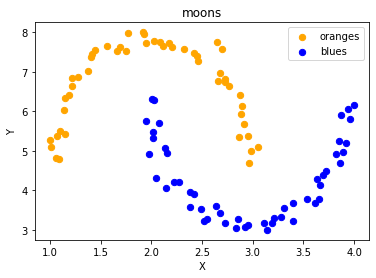import sklearn.datasets as ds
data, labels = ds.make_circles(n_samples=100,
shuffle=True,
noise=0.05,
random_state=None)

fig, ax = plt.subplots()

ax.scatter(data[labels==0, 0], data[labels==0, 1],
c='orange', s=40, label='oranges')
ax.scatter(data[labels==1, 0], data[labels==1, 1],
c='blue', s=40, label='blues')

ax.set(xlabel='X',
ylabel='Y',
title='circles')

ax.legend(loc='upper right')

Output:
<matplotlib.legend.Legend at 0x7f1c3d3caa30>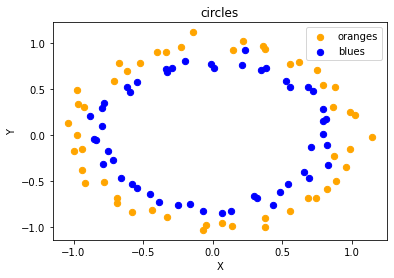print(__doc__)

import matplotlib.pyplot as plt

from sklearn.datasets import make_classification
from sklearn.datasets import make_blobs
from sklearn.datasets import make_gaussian_quantiles

plt.figure(figsize=(8, 8))

plt.subplot(321)
plt.title("One informative feature, one cluster per class", fontsize='small')
X1, Y1 = make_classification(n_features=2, n_redundant=0, n_informative=1,
n_clusters_per_class=1)
plt.scatter(X1[:, 0], X1[:, 1], marker='o', c=Y1,
s=25, edgecolor='k')

plt.subplot(322)
plt.title("Two informative features, one cluster per class", fontsize='small')
X1, Y1 = make_classification(n_features=2, n_redundant=0, n_informative=2,
n_clusters_per_class=1)
plt.scatter(X1[:, 0], X1[:, 1], marker='o', c=Y1,
s=25, edgecolor='k')

plt.subplot(323)
plt.title("Two informative features, two clusters per class",
fontsize='small')
X2, Y2 = make_classification(n_features=2,
n_redundant=0,
n_informative=2)
plt.scatter(X2[:, 0], X2[:, 1], marker='o', c=Y2,
s=25, edgecolor='k')

plt.subplot(324)
plt.title("Multi-class, two informative features, one cluster",
fontsize='small')
X1, Y1 = make_classification(n_features=2,
n_redundant=0,
n_informative=2,
n_clusters_per_class=1,
n_classes=3)
plt.scatter(X1[:, 0], X1[:, 1], marker='o', c=Y1,
s=25, edgecolor='k')

plt.subplot(325)
plt.title("Gaussian divided into three quantiles", fontsize='small')
X1, Y1 = make_gaussian_quantiles(n_features=2, n_classes=3)
plt.scatter(X1[:, 0], X1[:, 1], marker='o', c=Y1,
s=25, edgecolor='k')

plt.show()

Automatically created module for IPython interactive environment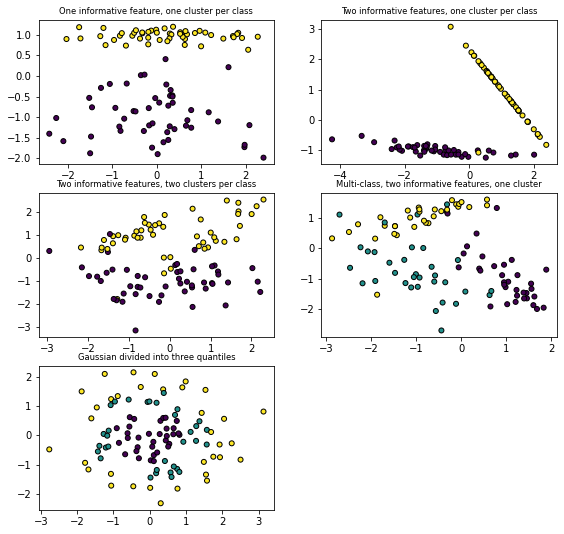### Exercises

#### Exercise 1

Create two clusters which look similar to this one: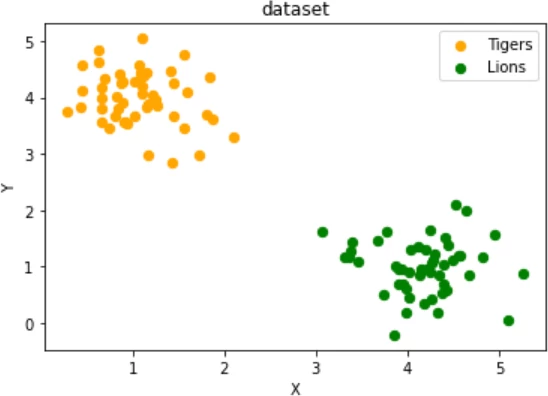two testsets which are separable with a perceptron without a bias node.

#### Exercise 2

Create two clusters similar to the following image: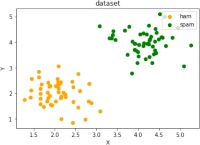#### Exercise 3

Create a dataset with five classes "Tiger", "Lion", "Penguin", "Dolphin", and "Python". The sets should look similar to the following diagram: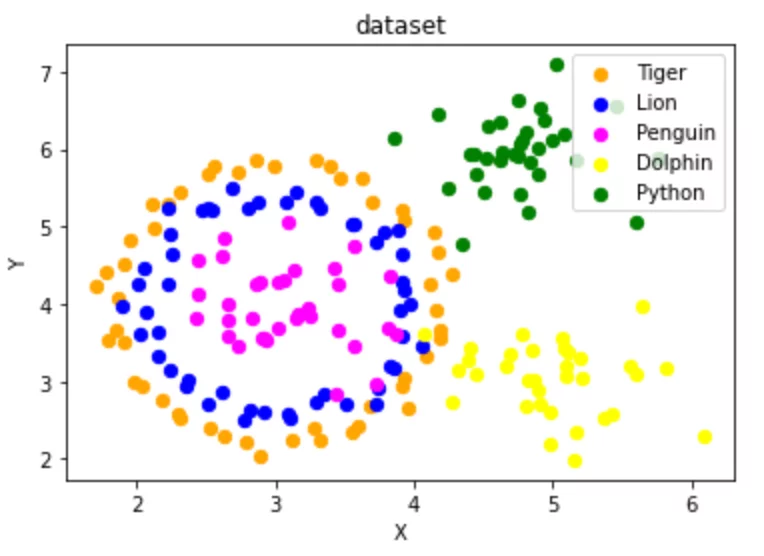### Solutions

#### Solution to Exercise 1

data, labels = make_blobs(n_samples=100,
cluster_std = 0.5,
centers=[[1, 4] ,[4, 1]],
random_state=1)

fig, ax = plt.subplots()

colours = ["orange", "green"]
label_name = ["Tigers", "Lions"]
for label in range(0, 2):
ax.scatter(data[labels==label, 0], data[labels==label, 1],
c=colours[label], s=40, label=label_name[label])

ax.set(xlabel='X',
ylabel='Y',
title='dataset')

ax.legend(loc='upper right')

Output:
<matplotlib.legend.Legend at 0x7f1c3d1a5400>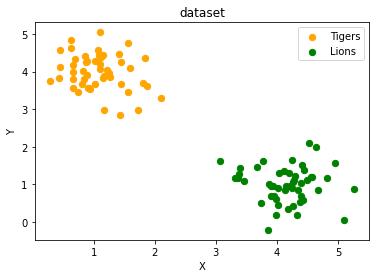#### Solution to Exercise 2

data, labels = make_blobs(n_samples=100,
cluster_std = 0.5,
centers=[[2, 2] ,[4, 4]],
random_state=1)

fig, ax = plt.subplots()

colours = ["orange", "green"]
label_name = ["ham", "spam"]
for label in range(0, 2):
ax.scatter(data[labels==label, 0], data[labels==label, 1],
c=colours[label], s=40, label=label_name[label])

ax.set(xlabel='X',
ylabel='Y',
title='dataset')

ax.legend(loc='upper right')

Output:
<matplotlib.legend.Legend at 0x7f1c401d3040>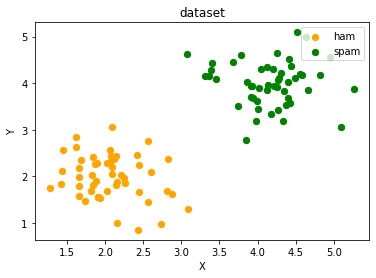#### Solution to Exercise 3

import sklearn.datasets as ds
data, labels = ds.make_circles(n_samples=100,
shuffle=True,
noise=0.05,
random_state=42)

centers = [[3, 4], [5, 3], [4.5, 6]]
data2, labels2 = make_blobs(n_samples=100,
cluster_std = 0.5,
centers=centers,
random_state=1)

for i in range(len(centers)-1, -1, -1):
labels2[labels2==0+i] = i+2

print(labels2)
labels = np.concatenate([labels, labels2])
data = data * [1.2, 1.8] + [3, 4]

data = np.concatenate([data, data2], axis=0)

[2 4 4 3 4 4 3 3 2 4 4 2 4 4 3 4 2 4 4 4 4 2 2 4 4 3 2 2 3 2 2 3 2 3 3 3 3
3 4 3 3 2 3 3 3 2 2 2 2 3 4 4 4 2 4 3 3 2 2 3 4 4 3 3 4 2 4 2 4 3 3 4 2 2
3 4 4 2 3 2 3 3 4 2 2 2 2 3 2 4 2 2 3 3 4 4 2 2 4 3]

fig, ax = plt.subplots()

colours = ["orange", "blue", "magenta", "yellow", "green"]
label_name = ["Tiger", "Lion", "Penguin", "Dolphin", "Python"]
for label in range(0, len(centers)+2):
ax.scatter(data[labels==label, 0], data[labels==label, 1],
c=colours[label], s=40, label=label_name[label])

ax.set(xlabel='X',
ylabel='Y',
title='dataset')

ax.legend(loc='upper right')

Output:
<matplotlib.legend.Legend at 0x7f1c402bc0d0>